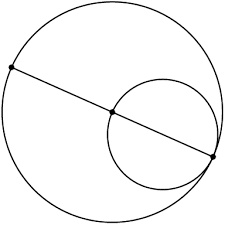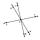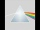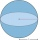# Prove

Prove that k1 and k2 are the equations of two circles. Find the equation of the line that passes through the centers of these circles.

k1: x2+y2+2x+4y+1=0
k2: x2+y2-8x+6y+9=0

Result

p = (Correct answer is: p=x+5y+11=0)#### Solution:We would be pleased if you find an error in the word problem, spelling mistakes, or inaccuracies and send it to us. Thank you!Tips to related online calculators
For Basic calculations in analytic geometry is a helpful line slope calculator. From coordinates of two points in the plane it calculate slope, normal and parametric line equation(s), slope, directional angle, direction vector, the length of segment, intersections the coordinate axes etc.
Looking for help with calculating roots of a quadratic equation?
Do you have a linear equation or system of equations and looking for its solution? Or do you have quadratic equation?
Pythagorean theorem is the base for the right triangle calculator.

#### You need to know the following knowledge to solve this word math problem:

We encourage you to watch this tutorial video on this math problem:

## Next similar math problems:

• Intersections 3Find the intersections of the circles x2 + y2 + 6 x - 10 y + 9 = 0 and x2 + y2 + 18 x + 4 y + 21 = 0
• Curve and lineThe equation of a curve C is y=2x² -8x+9 and the equation of a line L is x+ y=3 (1) Find the x co-ordinates of the points of intersection of L and C. (2) Show that one of these points is also the stationary point of C?
• Sphere from tree pointsEquation of sphere with three point (a,0,0), (0, a,0), (0,0, a) and center lies on plane x+y+z=a
• Linear systemSolve a set of two equations of two unknowns: 1.5x+1.2y=0.6 0.8x-0.2y=2
• Sphere equationObtain the equation of sphere its centre on the line 3x+2z=0=4x-5y and passes through the points (0,-2,-4) and (2,-1,1).
• Non linear eqsSolve the system of non-linear equations: 3x2-3x-y=-2 -6x2-x-y=-7
• Linsys2Solve two equations with two unknowns: 400x+120y=147.2 350x+200y=144
• Find the 15Find the tangent line of the ellipse 9 x2 + 16 y2 = 144 that has the slope k = -1
• The angle of linesCalculate the angle of two lines y=x-21 and y=-2x+14Which of the points belong function f:y= 2x2- 3x + 1 : A(-2, 15) B (3,10) C (1,4)
• EquationsSolve following system of equations: 6(x+7)+4(y-5)=12 2(x+y)-3(-2x+4y)=-44Please determine the solvability conditions of the equation, solve the equation and perform the test: x divided by x squared minus 2x plus1 the whole minus x + 3 divided by x squared minus 1 this is equal to 0: x/(x2-2x+1) - (x+3)/( x2-1) = 0Ellipse is expressed by equation 9x2 + 25y2 - 54x - 100y - 44 = 0. Find the length of primary and secondary axes, eccentricity, and coordinates of the center of the ellipse.X+y=5, find xy (find the product of x and y if x+y = 5)Find x, y, and z such that x³+y³+z³=k, for each k from 1 to 100. Write down the number of solutions.The line passed through three points - see table: x y -6 4 -4 3 -2 2 Write line equation in y=mx+b formFind the magnitude of the angle at which the ellipse x2 + 5 y2 = 5 is visible from the point P[5, 1] .